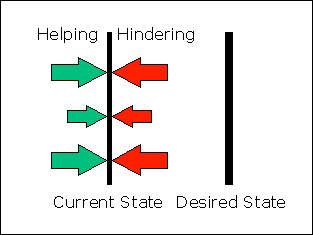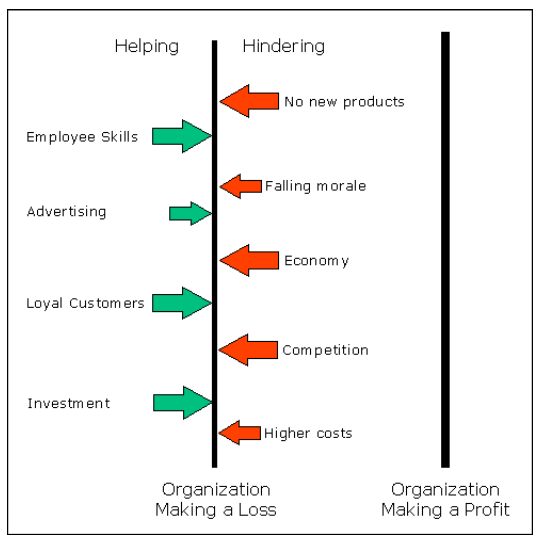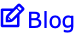MWLS.com

## Force-Field Analysis

Home | Articles | Productivity | Force-Field AnalysisDeveloped by the organizational researcher Kurt Lewin, the force-field diagram allows you to analyse the forces which help and hinder you from closing the gap between where you are now and where you want to be.

Because it deals with current and desired situations, force-field analysis is particularly useful in generating solutions in problem-solving and developing strategies for change management.

### Constructing a Force-Field Diagram

There are three steps in constructing a force-field diagram:

1. Draw a line down the centre of a flip chart page to represent the situation that currently exists.
2. Draw a second line, parallel to the first, on the right-hand side of the sheet to represent the desired situation
3. Now list the helping forces on the left of the centre line and the hindering forces on the right of the line.

The forces are identified by using problem-solving and data-collection techniques. You can also use Brainstorming to identify the forces, but make sure that you validate the results.

The 'forces' are often shown as arrows: the helping forces push the 'current state' towards the 'desired state' and the hindering forces push away from it. Representing the relative strength of the helping and hindering forces is often useful.

Some groups use arrows of different weights and sizes. Other groups use a scale (e.g. 5 = very strong, 4 = strong, 3 = medium, 2 = low and 1 = weak) to evaluate the relative impact of the forces. Paired Comparisons is a tool that is useful for evaluating the relative strength of forces which are not easily quantifiable.

### An Example of a Force-Field Diagram

The following is an example of a force-field diagram created for the problem of an organization which is currently making a loss and needs to make a profit.Studying the diagram suggests ways in which the 'current state' can be moved towards the 'desired state'. For example, you could consider:

• Increasing the number or strength of the helping forces.

But this might result in the hindering forces pushing back even harder. A better way would be to do this in combination with:

• Decreasing the number or strength of the hindering forces.

Even better would be to double the movement with the same amount of effort by:

• Converting hidden forces into helping forces.

Also bear in mind that:

• If the situation is stable, the helping forces balance the hindering forces.
• If the situation is getting worse, the hindering forces are greater than the helping forces
• If the situation is improving, the helping forces are stronger than the hindering forces.

270   127